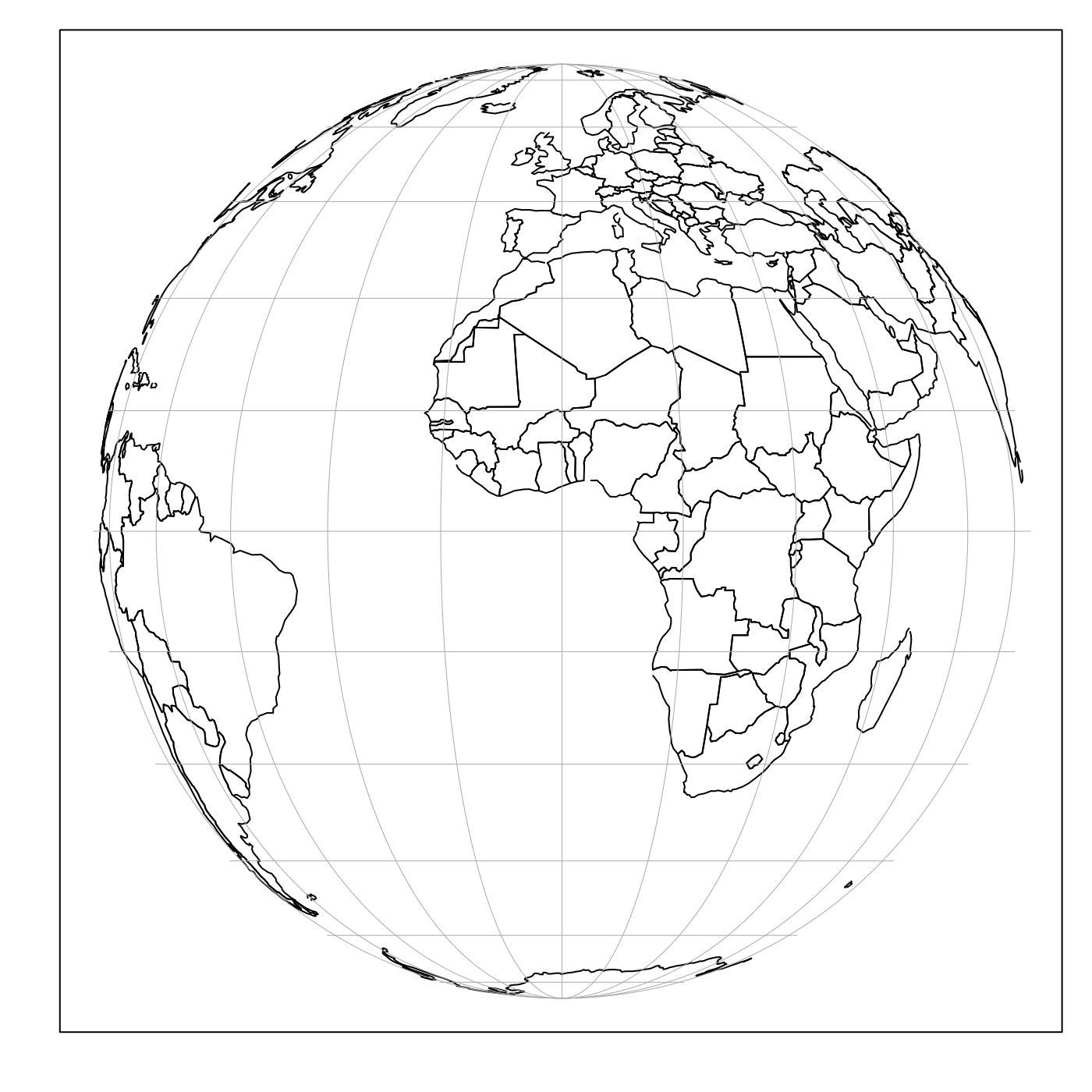Plot longitude and latitude grid on an existing map.

mapGrid(
dlongitude = 15,
dlatitude = 15,
longitude,
latitude,
col = "darkgray",
lty = "solid",
lwd = 0.5 * par("lwd"),
polarCircle = 0,
longitudelim,
latitudelim,
debug = getOption("oceDebug")
)

## Arguments

dlongitude increment in longitude, ignored if longitude is supplied, but otherwise determines the longitude sequence. increment in latitude, ignored if latitude is supplied, but otherwise determines the latitude sequence. numeric vector of longitudes, or NULL to prevent drawing longitude lines. numeric vector of latitudes, or NULL to prevent drawing latitude lines. color of lines line type line width a number indicating the number of degrees of latitude extending from the poles, within which zones are not drawn. optional argument specifying suggested longitude limits for the grid. If this is not supplied, grid lines are drawn for the whole globe, which can yield excessively slow drawing speeds for small-region plots. This, and latitudelim, are both set by mapPlot() if the arguments of the same name are passed to that function. similar to longitudelim. a flag that turns on debugging. Set to 1 to get a moderate amount of debugging information, or to 2 to get more.

## Details

This is somewhat analogous to grid(), except that the first two arguments of the latter supply the number of lines in the grid, whereas the present function has increments for the first two arguments.

## Plans

At the moment, the function cannot determine which lines might work with labels on axes, but this could perhaps be added later, making this more analogous with grid().

A map must first have been created with mapPlot().

Other functions related to maps: formatPosition(), lonlat2map(), lonlat2utm(), map2lonlat(), mapArrows(), mapAxis(), mapContour(), mapCoordinateSystem(), mapDirectionField(), mapImage(), mapLines(), mapLocator(), mapLongitudeLatitudeXY(), mapPlot(), mapPoints(), mapPolygon(), mapScalebar(), mapText(), mapTissot(), oceCRS(), shiftLongitude(), usrLonLat(), utm2lonlat()

## Examples

# \donttest{
library(oce)
data(coastlineWorld)
mapPlot(coastlineWorld, type='l', grid=FALSE,
longitudelim=c(-80, 10), latitudelim=c(0, 120),
projection="+proj=ortho")mapGrid(15, 15, polarCircle=15)# }